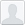# basemap meridians cut off

Hello,

I've been having a problem in my basemap plots of areas with small spatial
extents, where my meridians are cutoff like so:

I've tried simplifying the code as much as possible, but still get a similar
problem:
<http://matplotlib.1069221.n5.nabble.com/file/n41409/meridians_cutoff_atTop.png>

The meridians plot correctly when I use a normal mercator instead of the
transverse mercator projection, so switching to mercator is my last resort
option for now. Has anyone dealt with this problem before and found a
workaround?

Thank you!
-SeanPaul

Here's my simplified code:

import numpy as np
import matplotlib as mpl
from mpl_toolkits.basemap import Basemap
import matplotlib.pyplot as plt

"""Input desired lats (y) and lons(x) of plot"""

···

############
xmin = -166.22
xmax = -166.134

ymin = 53.7439
ymax = 53.7827
############

m = Basemap(projection='tmerc', lon_0=-165. , lat_0=0 , llcrnrlon=xmin,
llcrnrlat=ymin, urcrnrlon=xmax , urcrnrlat=ymax)

meridians = np.arange(xmin,xmax,(30/3600)) # 30 arcsecond spacing
m.drawmeridians(meridians)

parallels = np.arange(ymin,ymax,(30/3600))
m.drawparallels(parallels)

plt.show()

--
View this message in context: http://matplotlib.1069221.n5.nabble.com/basemap-meridians-cut-off-tp41409.html
Sent from the matplotlib - users mailing list archive at Nabble.com.spmls

July 5, 2013 10:58 AM

Hello,

I’ve been having a problem in my
basemap plots of areas with small spatial
extents, where my meridians are cutoff like so:

I’ve tried simplifying the code as much as possible, but still get a similarproblem:
The meridians plot correctly when I use a normal mercator instead of thetransverse mercator projection, so switching to mercator is my last resortoption for now. Has anyone dealt with this problem before and found aworkaround?Thank you!-SeanPaul

SeanPaul:
This looks like a bug in basemap - please file an issue on the github page ().-Jeff

···

https://github.com/matplotlib/basemap

Here’s my simplified code:

import numpy as np
import matplotlib as mpl
from mpl_toolkits.basemap import Basemap
import matplotlib.pyplot as plt

“”“Input
desired lats (y) and lons(x) of plot”""
############
xmin = -166.22
xmax = -166.134

ymin = 53.7439
ymax = 53.7827
############

m
= Basemap(projection=‘tmerc’, lon_0=-165. , lat_0=0 , llcrnrlon=xmin,
llcrnrlat=ymin,
urcrnrlon=xmax , urcrnrlat=ymax)

meridians = np.arange(xmin,xmax,(30/3600)) # 30 arcsecond spacing
m.drawmeridians(meridians)

parallels
= np.arange(ymin,ymax,(30/3600))
m.drawparallels(parallels)

plt.show()

View
this message in context: Sent
from the matplotlib - users mailing list archive at Nabble.com.------------------------------------------------------------------------------This
SF.net email is sponsored by Windows:Build for Windows Store._______________________________________________Matplotlib-users
mailing list

http://matplotlib.1069221.n5.nabble.com/basemap-meridians-cut-off-tp41409.html

http://p.sf.net/sfu/windows-dev2dev